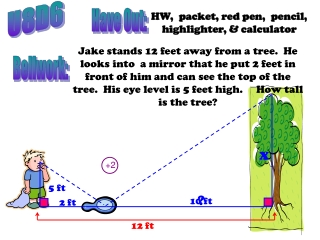DownloadDownload PresentationU8D6

# U8D6

Télécharger la présentation## U8D6

- - - - - - - - - - - - - - - - - - - - - - - - - - - E N D - - - - - - - - - - - - - - - - - - - - - - - - - - -
##### Presentation Transcript

1. U8D6 +2 Have Out: HW, packet, red pen, pencil, highlighter, & calculator Jake stands 12 feet away from a tree. He looks into a mirror that he put 2 feet in front of him and can see the top of the tree. His eye level is 5 feet high. How tall is the tree? Bellwork: x 5 ft ? 10 ft 2 ft 12 ft

2. Are the triangles similar? +1 +1 +1 +1 +1 +1 E AD and BE are  to AB A  B (Def of /Right s ) ACD  BCE ( of Incidence =  of Reflection) ACD ~ BCE (AA~ Thm) x D 5 ft 2 ft 10 ft B A C or or… The tree is 25 feet tall.

3. Work on S-56... you have 7 min. to complete it!

4. side length perimeter area figure a 1u 4u 1u2 figure b 2(1)=2u 8u 4u2 figure c 3(1)=3u figure d 4(1)=4u figure e 5(1)=5u S 56 fig. a fig. b fig. c fig. d fig. e 12u 9u2 16u2 16u 25u2 20u figure c to figure a figure d to figure a figure d to figure b Ratio of: Similarity Side lengths perimeters areas

5. S 56 c) Use the results from part (b) to write a summary of your observations about the relationships between side ratios, perimeter ratios, & area ratios in relation to the ratio of similarity. Look for a pattern & formulate your ideas into a conjecture. The side ratio & perimeter ratios are equal to the ratio of similarity (r). The area ratio is the square of the ratio of similarity.

6. For EACH set of similar figures, do all 3 tasks. S 57&58 • Calculate the area of each figure. • Write the ratio of the areas, small:large, and reduce. • Write the ratio of the shortest pair of corresponding sides, small:large, reduce if possible, and then square it. a) b) 4 3 5 10 6 8 12 24 A= ½ bh A= ½ bh A= ½ (5)(12) A= ½ (10)(24) A= ½ (3)(6) A= ½ (8)(4) = 30 u2 = 9 u2 = 120 u2 = 16 u2 ( ) 2 ( ) 2

7. For EACH set of similar figures, do all 3 tasks. S 57&58 • Calculate the area of each figure. • Write the ratio of the areas small:large and reduce. • Write the ratio of the shortest pair of corresponding sides, small:large, reduce if possible, and then square it. c) 9 4.5 10 20 A= bh A= (9)(20) A= (4.5)(10) = 180 u2 = 45 u2 ( ) 2

8. A= + A= + 30 15 6 12 4 10 10 25 d) 20 8 10 4 10 4 25 10 = (25•10)+ (½•10•20) = (4•10)+ (½•4•8) = 250 + 100 = 40 + 16 = 350 u2 = 56 u2 ( ) 2 e) The side ratio is the SAME as the ratio of similarity (r) & the area ratio is always the square of r!